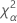# Methods and formulas for variance components in confidence intervals in Crossed Gage R&R Study

Select the method or formula of your choice.

## Common notation and rules for Gage R&R confidence intervals

For all variance components, lower and upper bounds for variance components must not be negative values. If the bounds calculated using the formulas are negative, then they are set to zero.

For all ratios between 0 and 1, lower and upper bounds should also be between 0 and 1. If the bounds are outside the range, they are set to 0 or 1 accordingly.

### Notation

TermDescriptionthe α *100 percentile of the chi-square distribution with nq degrees of freedom
Fα(nq, nγ)the α *100 percentile of the F distribution with nq and nγ degrees of freedom
Jthe number of operators
Ithe number of parts
Kthe number of replicates

For degrees of freedom:

Operators: n2=J–1

Parts: n1=J(1–I)

Replicates: n4=IJ(K–1)

MSOperator = S12

MSPart*Operator = S22

MSReplicates = S32

## Repeatability variance component confidence interval

Minitab calculates the lower and upper bounds for an exact (1 – α) *100% confidence interval. To calculate the one-sided confidence bounds, replace α/2 with α in H and G.

### Formulas

With operator
Without operator
Without interaction term

### Notation

TermDescriptionthe α *100 percentile of the chi-square distribution with nq degrees of freedom
Jthe number of operators
Ithe number of parts
Kthe number of replicates

## Reproducibility (or Operator) variance component confidence interval

Minitab uses the modified large-sample (MLS) method to calculate the lower and upper bounds for an approximate (1 – α) *100% confidence interval. To calculate the one-sided confidence bounds, replace α/2 with α in H and G.

### Formulas

With interaction termWithout interaction term### Notation

TermDescriptionthe α *100 percentile of the chi-square distribution with nq degrees of freedom
Jthe number of operators
Ithe number of parts
Kthe number of replicates

## Operator variance component confidence interval

Minitab uses the modified large-sample (MLS) method to calculate the lower and upper bounds for an approximate (1–α) *100% confidence interval. To calculate the one-sided confidence bounds, replace α/2 with α in H and G.

### Formulas### Notation

TermDescriptionthe α *100 percentile of the chi-square distribution with nq degrees of freedom
Jthe number of operators
Ithe number of parts
Kthe number of replicates

## Interaction variance component confidence interval

Minitab uses the modified large-sample (MLS) method to calculate the lower and upper bounds for an approximate (1 – α) *100% confidence interval. To calculate the one-sided confidence bounds, replace α/2 with α in H and G.

### Formulas### Notation

TermDescriptionthe α *100 percentile of the chi-square distribution with nq degrees of freedom
Jthe number of operators
Ithe number of parts
Kthe number of replicates

## Total gage variance component confidence interval

### With operator and interaction term

Minitab uses the modified large-sample (MLS) method to calculate the lower and upper bounds for an approximate (1 – α) *100% confidence interval. To calculate the one-sided confidence bounds, replace α/2 with α in H and G.### Without operator term

The lower and upper bounds for an exact (1 – α) *100% confidence interval are:### Without interaction term

Minitab uses the modified large-sample (MLS) method, the lower and upper bounds for an approximate (1 – α) *100% confidence interval. To calculate the one-sided confidence bounds, replace α/2 with α in H and G.### Notation

TermDescriptionthe α *100 percentile of the chi-square distribution with nq degrees of freedom
Jthe number of operators
Ithe number of parts
Kthe number of replicates

## Part-to-part variance component confidence interval

Minitab uses the modified large-sample (MLS) method to calculate the lower and upper bounds for an approximate (1 – α) *100% confidence interval. To calculate the one-sided confidence bounds, replace α/2 with α in H and G.

### With operator### Without operator### Without interaction### Notation

TermDescriptionthe α *100 percentile of the chi-square distribution with nq degrees of freedom
Jthe number of operators
Ithe number of parts
Kthe number of replicates

## Total variance component confidence interval

Minitab uses the modified large-sample (MLS) method to calculate the lower and upper bounds for an approximate (1 – α) *100% confidence interval. To calculate the one-sided confidence bounds, replace α/2 with α in H and G.

### With operator### Without operator### Without interaction term### Notation

TermDescriptionthe α *100 percentile of the chi-square distribution with nq degrees of freedom
Jthe number of operators
Ithe number of parts
Kthe number of replicates
By using this site you agree to the use of cookies for analytics and personalized content.  Read our policy